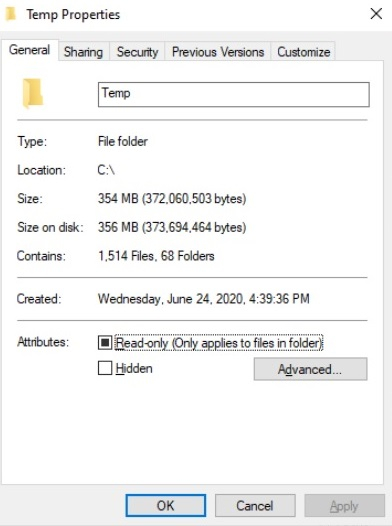# How to get the folder size using PowerShell?

We will first retrieve the content of the folder using the Get-ChildItem command and then pipeline the Measure-Object command as shown below.

Get-ChildItem C:\Temp\ -Recurse | Measure-Object -Property Length -Sum

## Output

Count : 1514
Average :
Sum : 372060503
Maximum :
Minimum :
Property : Length

So the above output shows that there is a total of 1514 files and folders and the sum shows the size of all the files and folders combined in KB. We can convert it to the MB as shown below.

(Get-ChildItem C:\Temp\ -Recurse | Measure-Object -Property Length -Sum).Sum / 1MB
354.824545860291

We can get the round figure,

[Math]::Round(
((Get-ChildItem C:\Temp\ -Recurse | Measure-Object -Property Length -Sum).Sum / 1MB),2
)
354.82

You can check the same on the folder path.Updated on: 30-Mar-2021

4K+ Views